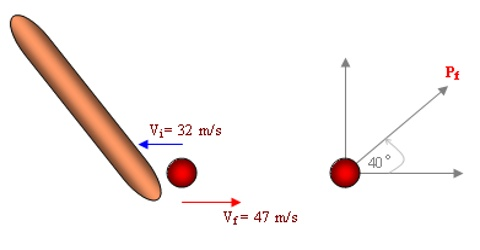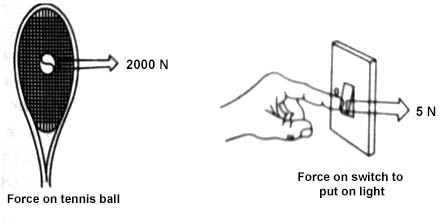Physics

# Impulsive ForceImpulsive Force

During collision, explosion, sudden strike etc such type of force acts. To strike carambole by a striker, hitting of a tennis ball by the bat, to kick a football, to hit a nail by a hammer, striking of a string of a musical instrument etc are a special type of force. It is called Impulsive force.

Impulsive force acts for such a brief period that during that time displacement of the body may be ignored. But as the magnitude of force is very large, so there is a sudden change of velocity and along with it momentum also changes. It is not possible to know or measure impulsive force correctly. It is not needed also. If change of momentum can be measured, i.e., if impulse of force is known, then total result of the force will be known. For this reason, this type of force is called impulsive force. That means, for a very brief period if a large force acts on a body, then that force is called impulsive force.

Example: Suppose, a tennis ball is struck to a racket then a strong torte acts on the tennis ball. Here the time of collision between the ball and the racket is very small.This type of force is an impulsive force [Fig. (a)]. Again, when the electric switch is made off’ and on, this impulsive force also becomes effective [Fig. (b)].

Again a product of a force and the duration of action of the force are called impulse of the force.

If force F is acting on a body for time t, then,

the impulse of force, J = F x t

= [(mv – mv0) / t] x t = [(mv – mv0) = change of momentum

So, Impulse of force is equal to the change of momentum.